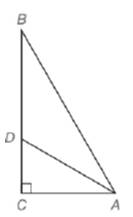Chapter 5.6, Problem 20EElementary Geometry For College St...

7th Edition
Alexander + 2 others
ISBN: 9781337614085

Solutions

Chapter
SectionElementary Geometry For College St...

7th Edition
Alexander + 2 others
ISBN: 9781337614085
Textbook Problem

Given: In Δ A B C , A D → bisects ∠ B A C A B = 20 and A C = 16Find: D C and D B

To determine

To find:

DC and DB.

Explanation

Given:

In ΔABC, AD bisects BAC, AB=20,AC=16.

Theorem used:

Angle bisector theorem:

If a ray bisects one angle of a triangle, then it divides the opposite side into segments whose lengths are proportional to the lengths of the two sides that form the bisected angle.

Calculation:

Given: AD bisects BAC.

Then by angle-bisector theorem, the ray AD divides the opposite side into segments whose lengths are proportional to the lengths of the two sides that form the bisected angle.

ABAC=BDDC.

Since ABC is a right triangle, by Pythagoras theorem we get

(AB)2=(AC)2+(BC)2

We have AB=20,AC=16.

(AB)2=(AC)2+(BC)2(20)2=(16)2+(BC)2400256=(BC)2144=(BC)212=BC

We have ABAC=BDDC

Still sussing out bartleby?

Check out a sample textbook solution.

See a sample solution

The Solution to Your Study Problems

Bartleby provides explanations to thousands of textbook problems written by our experts, many with advanced degrees!

Get Started

Fill in the blanks. 8. a. The general form of an equation of a line is_______.

Applied Calculus for the Managerial, Life, and Social Sciences: A Brief Approach

Evaluate the expression sin Exercises 116. (14)2

Finite Mathematics and Applied Calculus (MindTap Course List)

Which vector at the right could be a gradient for f(x, y)? a b c d

Study Guide for Stewart's Multivariable Calculus, 8th